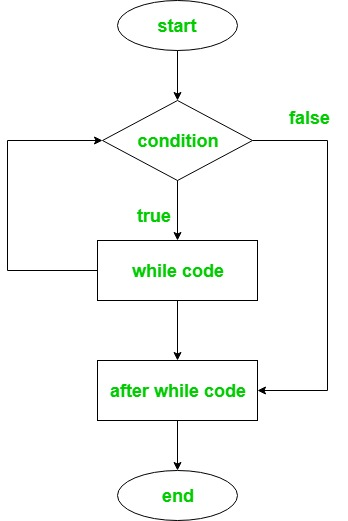GFG App
Open AppBrowser
Continue

# Ruby | Loops (for, while, do..while, until)

Looping in programming languages is a feature which clears the way for the execution of a set of instructions or functions repeatedly when some of the condition evaluates to true or false. Ruby provides the different types of loop to handle the condition based situation in the program to make the programmers task simpler. The loops in Ruby are :

while Loop

The condition which is to be tested, given at the beginning of the loop and all statements are executed until the given boolean condition satisfies. When the condition becomes false, the control will be out from the while loop. It is also known as Entry Controlled Loop because the condition to be tested is present at the beginning of the loop body. So basically, while loop is used when the number of iterations is not fixed in a program.
Syntax:

```while conditional [do]

# code to be executed

end```

Note: A while loop’s conditional is separated from code by the reserved word do, a newline, backslash(\), or a semicolon(;).
Flowchart:Example:

## Ruby

 `# Ruby program to illustrate 'while' loop`   `# variable x` `x = ``4`   `# using while loop ` `# here conditional is x i.e. 4` `while` `x >= ``1`   `# statements to be executed` `  ``puts ``"GeeksforGeeks"` `  ``x = x - ``1` `  `  `# while loop ends here` `end`

Output:

```GeeksforGeeks
GeeksforGeeks
GeeksforGeeks
GeeksforGeeks```

for Loop

for” loop has similar functionality as while loop but with different syntax. for loop is preferred when the number of times loop statements are to be executed is known beforehand. It iterates over a specific range of numbers. It is also known as Entry Controlled Loop because the condition to be tested is present at the beginning of the loop body.
Syntax:

```for variable_name[, variable...] in expression [do]

# code to be executed

end```

for: A special Ruby keyword which indicates the beginning of the loop.
variable_name: This is a variable name that serves as the reference to the current iteration of the loop.
in: This is a special Ruby keyword that is primarily used in for loop.
expression: It executes code once for each element in expression. Here expression can be range or array variable.
do: This indicates the beginning of the block of code to be repeatedly executed. do is optional.
end: This keyword represents the ending of ‘for‘ loop block which started from ‘do‘ keyword.
Example 1:

## Ruby

 `# Ruby program to illustrate 'for' ` `# loop using range as expression`   `i = ``"Sudo Placements"`   `# using for loop with the range` `for` `a ``in` `1``..``5` `do` `    `  ` ``puts i` ` `  `end`

Output:

```Sudo Placements
Sudo Placements
Sudo Placements
Sudo Placements
Sudo Placements```

Output:

```1
2
3
4
5```

Explanation: Here, we have defined the range 1..5. Range Operators create a range of successive values consisting of a start, end, and range of values in between. The (..) creates a range including the last term. The statement for a in 1..5 will allow a to take values in the range from 1 to 5 (including 5).
Example 2:

## Ruby

 `# Ruby program to illustrate 'for' ` `# loop using array  as expression`   `# array` `arr = [``"GFG"``, ``"G4G"``, ``"Geeks"``, ``"Sudo"``]`   `# using for loop` `for` `i ``in` `arr ``do` `    `  ` ``puts i` ` `  `end`

Output:

```GFG
G4G
Geeks
Sudo```

do..while Loop

do while loop is similar to while loop with the only difference that it checks the condition after executing the statements, i.e it will execute the loop body one time for sure. It is a Exit-Controlled loop because it tests the condition which presents at the end of the loop body.
Syntax:

```loop do

# code to be executed

break if Boolean_Expression

end```

Here, Boolean_Expression will result in either a true or false output which is created using comparing operators (>, =, <=, !=, ==). You can also use multiple boolean expressions within the parentheses (Boolean_Expressions) which will be connected through logical operators (&&, ||, !).
Example:

## Ruby

 `# Ruby program to illustrate 'do..while'loop`   `# starting of do..while loop` `loop ``do` `    `  ` ``puts ``"GeeksforGeeks"` ` `  ` ``val = ``'7'` ` `  ` ``# using boolean expressions` ` ``if` `val == ``'7'` `  ``break` ` ``end` ` `  `# ending of ruby do..while loop ` `end`

Output:

`GeeksforGeeks`

until Loop

Ruby until loop will executes the statements or code till the given condition evaluates to true. Basically it’s just opposite to the while loop which executes until the given condition evaluates to false. An until statement’s conditional is separated from code by the reserved word do, a newline, or a semicolon.
Syntax:

```until conditional [do]

# code to be executed

end```

Example:

## Ruby

 `# Ruby program to illustrate 'until' loop`   `var = ``7`   `# using until loop` `# here do is optional` `until` `var == ``11` `do`   `  ``# code to be executed` `  ``puts var * ``10` `  ``var = var + ``1` `  `  `# here loop ends` `end`

Output:

```70
80
90
100```

My Personal Notes arrow_drop_up